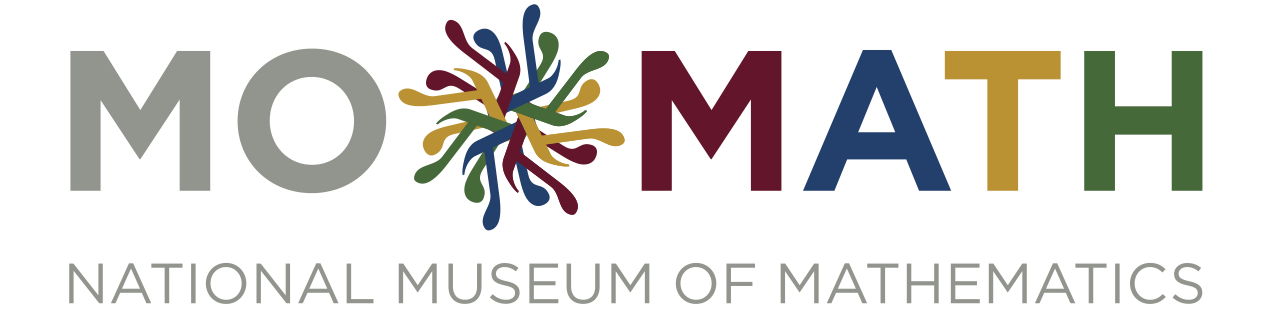##There always seem to be lots of boxes around this time of year. So that’s the theme of this week’s problems.
[asciimathsf]

### Boxes not Urns

Emerson is tagging boxes, using red, yellow, green and blue tags. Toward the end of the tagging, Emerson reaches into the box of remaining tags, and pulls out two completely at random. The digits of answer (B) to last week’s Champion Countdown problem give you the number of red, yellow, green and blue tags, respectively, just before Emerson pulls the two tags out. (Add leading zeros if necessary to bring that answer (B) up to four digits.)

What is the probability that the two tags are different colors?

### Boxes Nest

Charlie is going to make a homemade nesting boxes toy, by painting some boxes bright colors. Any size box is available, as long as all measurements are a whole number of inches. Charlie’s smallest box will be 1×1×1, the largest will be 8×8×8, and there will be four boxes in between. Each box doesn’t have to be a square—the length and width can vary—and while the tops of all of the boxes have to be facing up, boxes can be rotated if need be. So in making this toy, a 2×3×4 box is the same as a 2×4×3 box — you can tell the height apart from the other two dimensions, but you can’t distinguish length from width, because of the possibility of rotating the box. One box will fit in another only if there’s a way of orienting them such that each measurement of the outer box is strictly larger than the corresponding measurement of the inner box. For example, a 2×3×4 box fits in a 5×4×6 box but not in a 3×4×4 box. For the toy, the 1×1×1 box should fit in the next smallest, which fits in the next one, and so on, with the second largest fitting in the 8×8×8.

How many different sequences of box sizes might Charlie use for the set of nesting boxes?

## Solutions to Week 14

Crooked Clock. First, we only need to look for such times from noon to midnight; we can then double that number to get the final answer, since the clock precisely repeats itself every twelve hours. Next, if there are two right angles, then either the hour and minute hands overlap, point in opposite directions, or form a right angle. Because the hour hand makes one revolution in twelve hours and the minute hand makes twelve, the minute hand overtakes the hour hand eleven times in each twelve-hour period. Similarly, there are eleven times when the hour and minute hands point in opposite directions, eleven times when the minute hand is 90 degrees past the hour hand, and eleven times when the minute hand is 90 degrees before the hour hand. Since the motion of the clock is uniform, each of these events occurs every 12/11 of an hour. Thus, the hour and minute hands overlap at noon, 1/11 of an hour after 1 pm, 2/11 of an hour after 2pm and so on up to 10/11 of an hour after 10 pm. 1/11 of an hour is 60/11 minutes, which is 5 minutes and 5/11 of a minute. Hence at noon, the second hand is in the same place as the matching hour and minute hands, at 1/11 of an hour after 1 pm the second hand is 5/11 of the way around the clock face, at 2/11 of an hour the second hand is 10/11 of the way around the clock face, and so on. The point is that at each one of these times except noon, the second hand is some number of elevenths of the way around from the matching hour and minute hands. But to make a right angle, it would have to be 1/4 or 3/4 of the way around. No number of elevenths is equal to a whole number of quarters, and at noon all three hands line up, so there are no configurations of the type sought when the minute hand and hour hand line up.

We can now proceed with the other configurations similarly. The minute and hour hands are opposite each other precisely at 6pm, and at that time the second hand is lined up with the minute hand, so that does not provide a solution; and at all the other times when the minute and hour hands are opposite each other, the minute and second hands differ by some number of elevenths. So there are none of the desired configurations when the minute and hour hands point in opposite directions.

At 3pm and 9pm exactly, the minute and hour hands are at right angles; and the second hand lines up precisely with the minute hand at these times, so it also makes a right angle with the hour hand. So these times work. As in the first case, though, at all other times that the minute and hour hands are at right angles, the second hand differs from their positions by some number of elevenths of a turn, not 1/4 of a turn or 3/4 of a turn. Hence, 3pm and 9pm are the only two pm times that work, and adding 3am and 9am, we get a total of 4 times per day that you can form two right angles with the hands.

## Recent Weeks

Links to all of the puzzles and solutions are on the Complete Varsity Math page.

Come back next week for answers and more puzzles.